Therorems

Chapter 10 Class 10 Circles
Serial order wise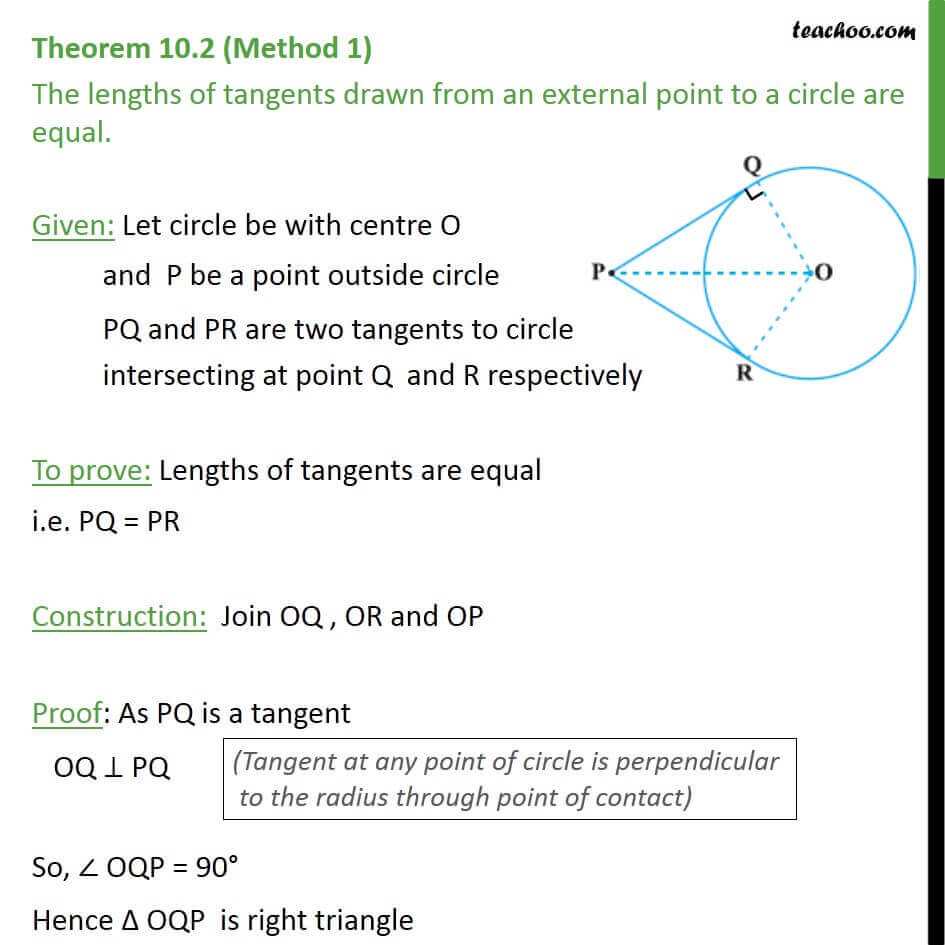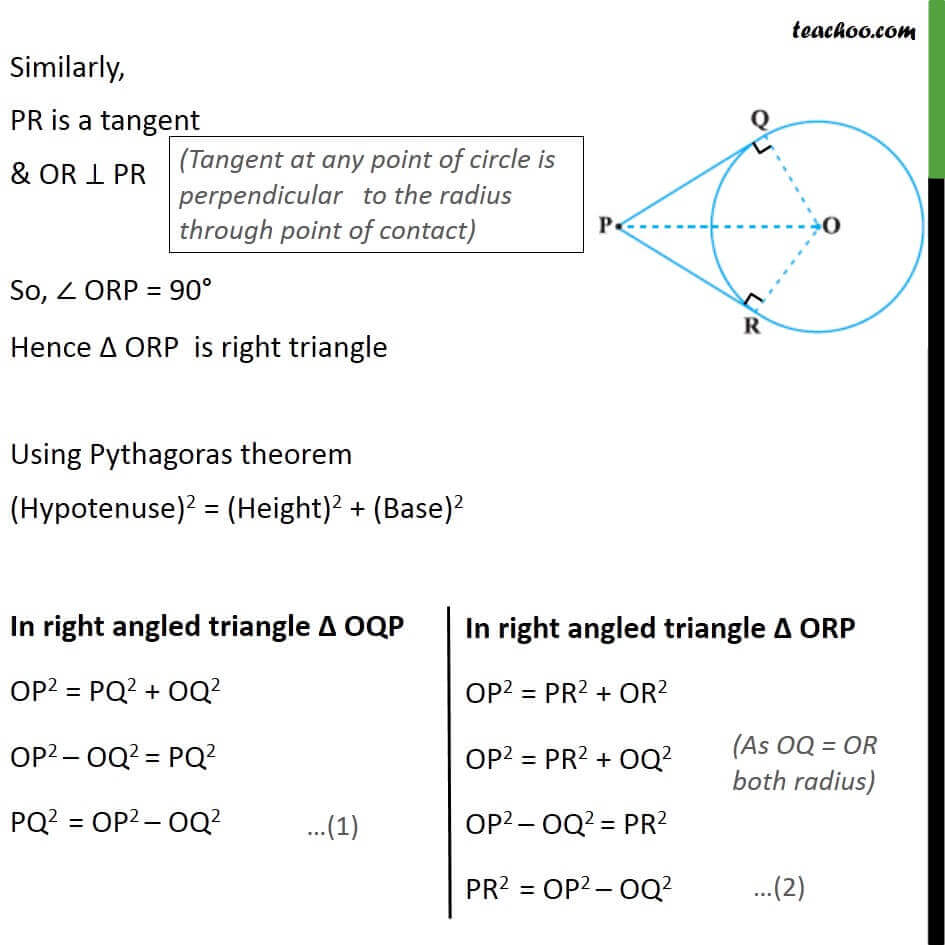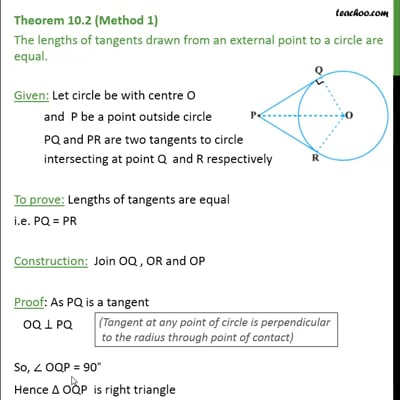This video is only available for Teachoo black users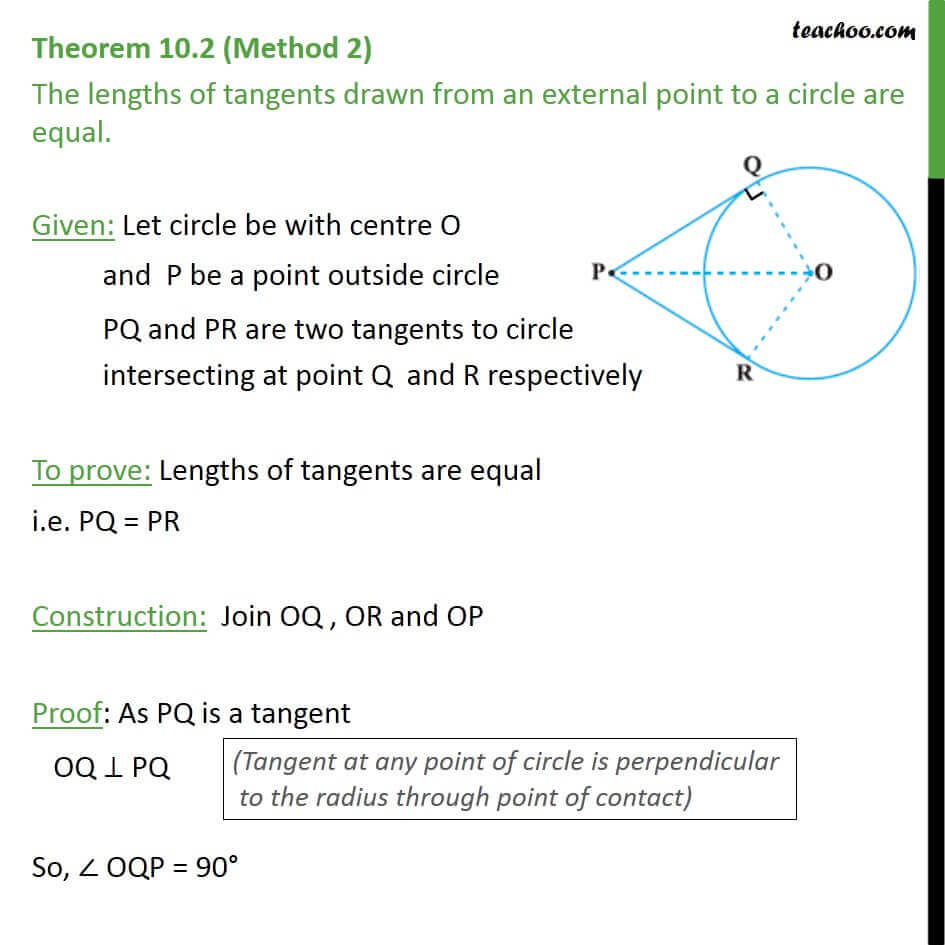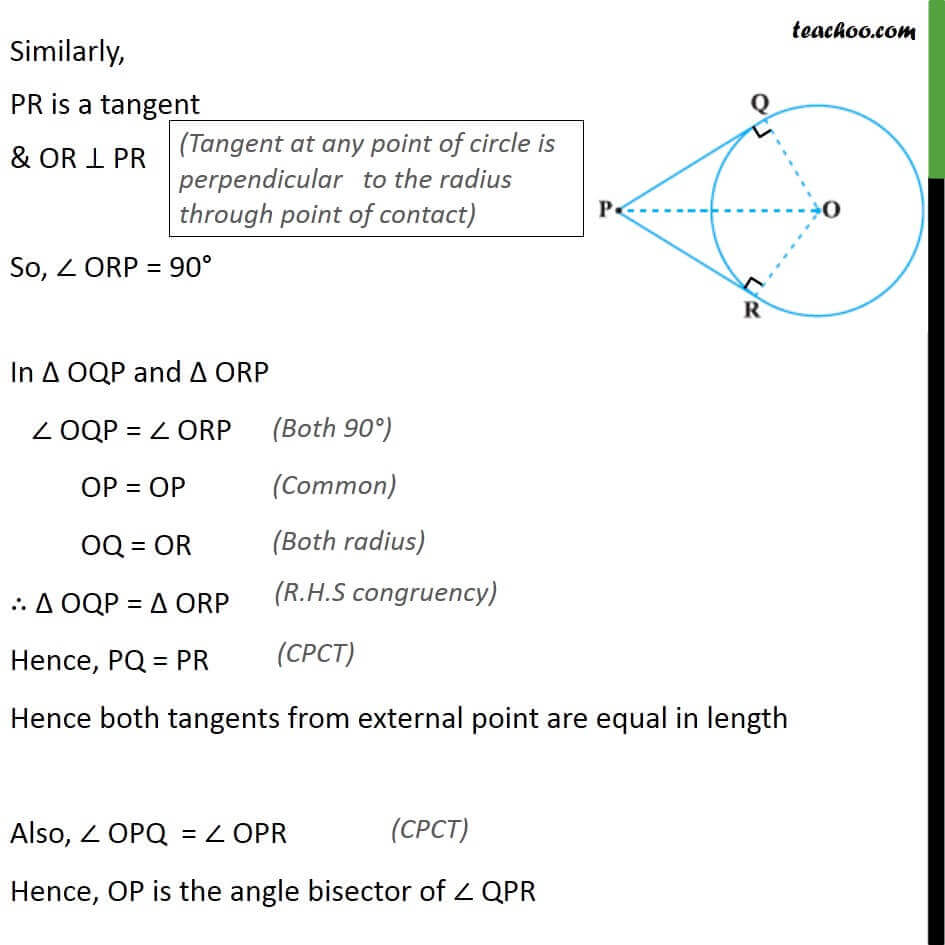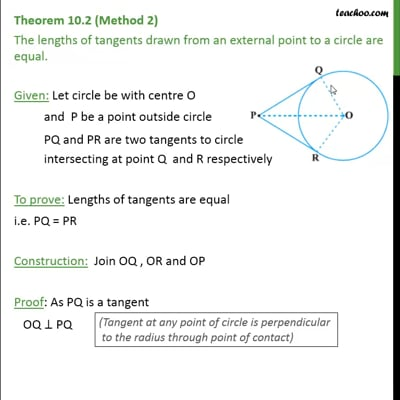This video is only available for Teachoo black users

Maths Crash Course - Live lectures + all videos + Real time Doubt solving!

### Transcript

Theorem 10.2 (Method 1) The lengths of tangents drawn from an external point to a circle are equal. Given: Let circle be with centre O and P be a point outside circle PQ and PR are two tangents to circle intersecting at point Q and R respectively To prove: Lengths of tangents are equal i.e. PQ = PR Construction: Join OQ , OR and OP Proof: As PQ is a tangent OQ ⊥ PQ So, ∠ OQP = 90° Hence Δ OQP is right triangle Similarly, PR is a tangent & OR ⊥ PR So, ∠ ORP = 90° Hence Δ ORP is right triangle Using Pythagoras theorem (Hypotenuse)2 = (Height)2 + (Base)2 From (1) and (2) PQ2 = PR2 PQ = PR Hence proved Theorem 10.2 (Method 2) The lengths of tangents drawn from an external point to a circle are equal. Given: Let circle be with centre O and P be a point outside circle PQ and PR are two tangents to circle intersecting at point Q and R respectively To prove: Lengths of tangents are equal i.e. PQ = PR Construction: Join OQ , OR and OP Proof: As PQ is a tangent OQ ⊥ PQ So, ∠ OQP = 90° Similarly, PR is a tangent & OR ⊥ PR So, ∠ ORP = 90° In Δ OQP and Δ ORP ∠ OQP = ∠ ORP OP = OP OQ = OR ∴ Δ OQP = Δ ORP Hence, PQ = PR Hence both tangents from external point are equal in length Also, ∠ OPQ = ∠ OPR Hence, OP is the angle bisector of ∠ QPR